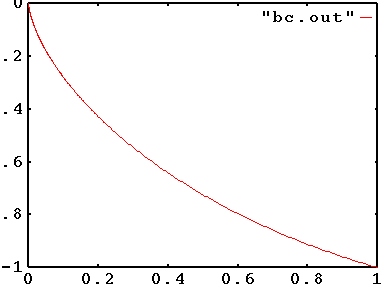## Brachistochrone Problem

The problem is to design a frictionless ramp that connects (0,0) to (1,1) (the positive y-axis is assumed to point downward) such that a ball rolling down it will get to (1,1) in the shortest amount of time.

We give three formulations. Each formulation involves a discretization of an integral that represents time.

`bc4.mod` is the formulation found in most books (see, e.g., the classical text on calculus by Thomas). This version involves minimizing an integral of a nonconvex function of the unknown function y(x) giving the height (or depth really) of the ramp as a function of horizontal position. This formulation is a difficult nonconvex optimization problem.

In `bc7.mod`, the roles of x and y are interchanged (we assume monotonicity of the function y(x)). That is, we seek a function x(y) that gives the horizontal position of the ramp as it crosses level y. This version turns out to be convex. It can also be cast as a second-order cone optimization problem.

`bc5.mod` is the most straightforward of the models. In it, x(t) and y(t) are both unknown functions to be determined. They must satisfy the boundary conditions and the force balance equations. The calculation of the time for a ramp so given is then easy to specify.

It is interesting to experiment with the spacing of the discretization of the independent variable (x in `bc4.mod` and y in `bc7.mod`). Uniform spacing leads to very slow convergence. Appropriate nonuniform spacing gives dramatically better results. See the model files for examples.

The plot shown below was produced with `gnuplot` using the following command:

``` plot "bc.out" w lines ```